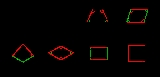xOverview

In Euclidean plane geometry
Euclidean geometry
Euclidean geometry is a mathematical system attributed to the Alexandrian Greek mathematician Euclid, which he described in his textbook on geometry: the Elements. Euclid's method consists in assuming a small set of intuitively appealing axioms, and deducing many other propositions from these...

, a quadrilateral is a polygon
Polygon
In geometry a polygon is a flat shape consisting of straight lines that are joined to form a closed chain orcircuit.A polygon is traditionally a plane figure that is bounded by a closed path, composed of a finite sequence of straight line segments...

with four sides (or 'edges') and four vertices or corners. Sometimes, the term quadrangle is used, by analogy with triangle
Triangle
A triangle is one of the basic shapes of geometry: a polygon with three corners or vertices and three sides or edges which are line segments. A triangle with vertices A, B, and C is denoted ....

, and sometimes tetragon for consistency with pentagon
Pentagon
In geometry, a pentagon is any five-sided polygon. A pentagon may be simple or self-intersecting. The sum of the internal angles in a simple pentagon is 540°. A pentagram is an example of a self-intersecting pentagon.- Regular pentagons :In a regular pentagon, all sides are equal in length and...

(5-sided), hexagon (6-sided) and so on. The word quadrilateral is made of the words quad (meaning "four") and lateral (meaning "of sides").

The origin of the word quadrilateral is from the two Latin words "quadri", a variant of four, and "latus" meaning side.

Simple polygon
In geometry, a simple polygon is a closed polygonal chain of line segments in the plane which do not have points in common other than the common vertices of pairs of consecutive segments....

(not self-intersecting) or complex
Complex polygon
The term complex polygon can mean two different things:*In computer graphics, as a polygon which is neither convex nor concave.*In geometry, as a polygon in the unitary plane, which has two complex dimensions.-Computer graphics:...

(self-intersecting), also called crossed.How can I learn quadrilateral angles easierAre all recatngles parallelograms and why?Have all quadrilaterals got 4 right angles?What is the most right angles a triangle can have? What is the fewest number of right angles a triangle can have?What do the angels equal in an irregular quadrilateral?A quadrilateral with two congruent opposite angles and two congruent adjacent sides?DiscussionsQualrilateral with two sets of parallel sides"if in a quadrilateral, each pair of opposite angles is equal,then it is a parallelogram" i want the proof of this theorammaths